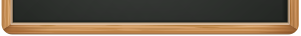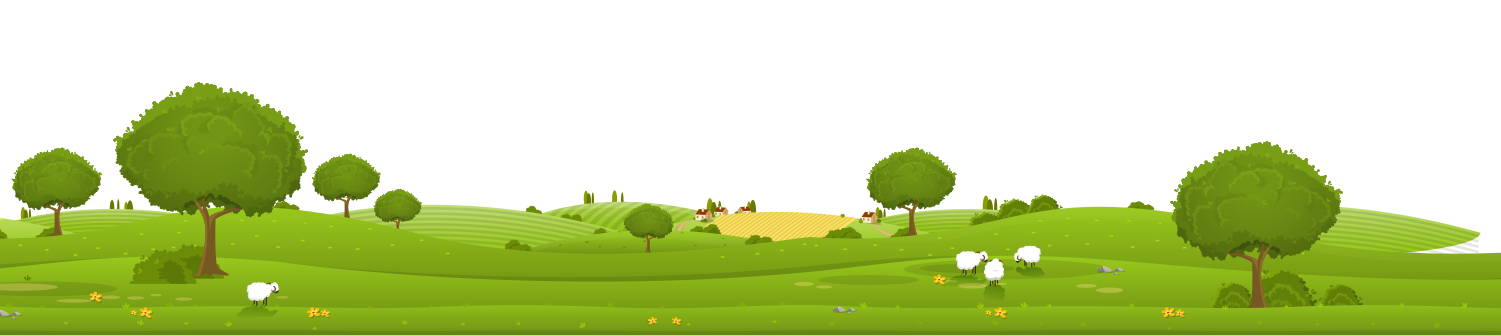Maths

Autumn Term

This term in Maths we will be focussing on Number and the Four operations so that we are ready to apply these skills to other areas of Maths later in the year.

We will begin by exploring place value (up to 4 digits) in order to get a real solid understanding of numbers and how they work. We will practise making numbers with concrete resources, using place value grids and partioning numbers into thousands, hundreds, tens and one. We will practise counting forwards and backwards in various different steps (e.g. counting in 50s) and we will explore negative numbers. We will also be looking at finding 10, 100 or 1000 more or less than a given number and rounding numbers to the nearest 10, 100 or 1000.

Next we will be moving onto methods for addition and subtraction, including both column methods and mental strategies. We will also be learning to estimate and use inverse operations to work backwards and check our answers.

Throughout all of this, as well as gaining fluency, we will be applying our skills to reasoning problems and problem solving activities.

Spring Term

This term, we will be looking at multiplication and division, again focussing on mental and written methods. We will be learning to derive multiplication and division facts from those that we already know and find factor pairs. We will also practise using the grid method, formal column methods and the bus stop method to help us when working with bigger numbers. It really helps at this point to be fairly confident with times tables so please encourage your child to practise at home!

After that, we will be exploring fractions and decimals. We will be finding fractions of shapes, objects and numbers as well as investigating equivalent fractions. We will also be learning to add and subtract fractions within 1 whole. As part of our fractions and decimals learning, we will be exploring the tenths and hundreths columns in place value and how these relate to decimal numbers.

Summer Term

This term, we will be looking at measure, starting with time. We will look at telling the time, with both analogue and digital clocks, whilst also thinking about how we measure time and how we can calculate the duration of activities.

We will then be looking at money, which will involve lots of practical adding and subtracting alongside lots of problem solving and real-life, practical problems.

We will also be looking at measure in terms of length, mass and capacity. We will be thinking about which equipment we will need and how we can measure accurately as well as putting our calculation skills to the test to answer lots of measurement related problems. During our work with measure, we will also be learning about perimeter and area

Finally, we will be thinking about angles (right, acute and obtuse), lines (vertical, horizontal, parallel and perpendicular) and shapes (2D and 3D)What's on

Keep up to date and find out what's happening in your schoolCalendar